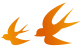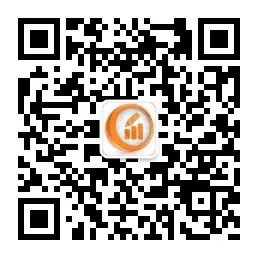# Python数分实战｜从六个视角分析了58万条电商用户行为数据

## 数据预处理

``# 读入数据``sales_df = pd.read_excel('./Online Retail.xlsx', sheet_name='Online Retail')``# 列名重命名``sales_df.rename(columns={'InvoiceDate': 'InvoiceTime'}, inplace=True)``# 删除重复值``rows_before = sales_df.shape``sales_df.drop_duplicates(inplace=True)``rows_after = sales_df.shape``# 填充空值``sales_df['CustomerID'].fillna('0', inplace=True)``# 增添新列``sales_df['InvoiceTime'] = pd.to_datetime(sales_df['InvoiceTime'], errors='coerce')``sales_df['Date'] = pd.to_datetime(sales_df['InvoiceTime'].dt.date, errors='coerce')``sales_df['Month'] = sales_df['InvoiceTime'].dt.month``# 数据类型转换``sales_df['Quantity'] = sales_df['Quantity'].astype('int32')``sales_df['UnitPrice'] = sales_df['UnitPrice'].astype('float')``sales_df['CustomerID'] = sales_df['CustomerID'].astype('int32')``sales_df['InvoiceNo'] = sales_df['InvoiceNo'].astype('str')``# 计算总价``sales_df['SumPrice'] = sales_df['Quantity'] * sales_df['UnitPrice']``# 拆分订单``query_c = sales_df['InvoiceNo'].str.contains('C')``# 只含取消订单``sales_cancel = sales_df.loc[query_c, :].copy()``# 只含成功订单``sales_success = sales_df.loc[-query_c, :].copy()``# 增加原订单号``sales_cancel['SrcInvoiceNo'] = sales_cancel['InvoiceNo'].str.split('C', expand=True)``new_data = pd.merge(sales_cancel, sales_success, left_on='SrcInvoiceNo',right_on='InvoiceNo')``query_free = sales_success['UnitPrice'] == 0``# 只含免费订单``sales_free = sales_success.loc[query_free, :].copy()``# 只含普通订单``sales_success = sales_success.loc[-query_free, :]``query_minus = sales_success['UnitPrice'] < 0``sales_success = sales_success.loc[-query_minus, :]``

## 数据分析及可视化

### 1、销售情况的描述性统计

#### 1.3 时间维度

2011年12月的前8天基本延续了11月下旬的销售趋势，但在12月9日订单量大幅下降时，却创造了样本区间内销量和销售额的历史新高。说明存在某笔或某几笔购买量极大的订单，从而使得销售额大幅上升。

## 小结

#### 推荐阅读End▲长按加微信群▲长按关注公众号